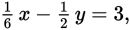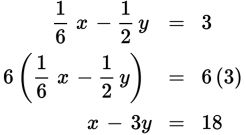# SAT Math Multiple Choice Question 280: Answer and Explanation

### Test Information

Question: 280

10. Ifwhat is the value of x – 3y?

• A. 6
• B. 12
• C. 18
• D. 36

Explanation:

C

Difficulty: Medium

Category: Heart of Algebra / Linear Equations

Strategic Advice: Only one equation is given, and it has two variables. This means that you don't have enough information to solve for either variable. Instead, look for the relationship between the variable terms in the equation and those in the expression that you are trying to find, x - 3y.

Getting to the Answer: The expression that you're looking for doesn't have fractions, so clear the fractions in the equation by multiplying both sides by 6. Don't forget to distribute the 6 to both terms on the left side of the equation.This yields the expression that you are looking for, x - 3y, so no further work is required-just read the value on the right-hand side of the equation. The answer is 18, which is (C).